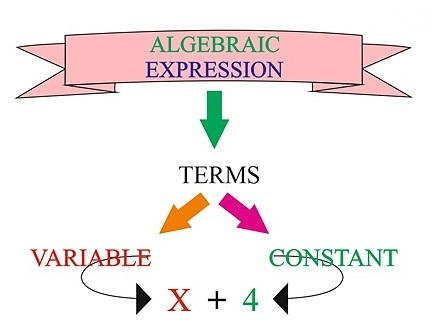# What are Algebraic Expressions?

Algebraic Expressions are used to calculate solutions for any Mathematical operations that include variables such as addition, subtraction, multiplication, or division. Any unknown value is represented as the letters x,y, and z in the fundamentals of Algebra. These letters are referred to as variables. An algebraic expression can have both variables and constants.(Source = Embibe.com)

There are three types of algebraic expressions:

1. Monomial Expression
2. Binomial Expression
3. Polynomial Expression

Let’s assume that A and B are playing with sticks and have thought of forming a number pattern. A takes four sticks and forms a number 4, B added three more sticks to form a pattern with two 4’s. Both A and B realize that if they keep adding 3 sticks in each round, they can make one extra four. We can now deduce that they need 4+ 3(n-1) sticks, in general, to make a pattern with n number of 4’s. The expression “4+3(n-1)” is called an algebraic expression.

## What are Algebraic Expressions?

Many students wonder what exactly is algebraic expressions? or what is the definition of algebraic expressions? The combination of the constants and the variables connected by some or all of the four fundamental operations addition $\left(+\right),$ subtraction $\left(–\right),$ multiplication $\left(×\right),$ and division $\left(÷\right)$ is known as an algebraic expression.(Source = Embibe.com)

## Constant

Any quantity whose value never changes in the given subject (or boundary) of discussion is called a constant.

## Variable

Any quantity whose value changes in the given subject (or boundary) of discussion is called a variable.

## Terms of Algebraic Expressions

In an algebraic expression, a term may consist of $\left(i\right)$ only constant, $\left(ii\right)$ only one variable, $\left(iii\right)$ product of two or more variables,$\left(iv\right)$ a product of both the variable $\left(s\right)$ and the constant part. The terms may be positive or negative.

## Coefficients

The fixed (or constant) number part along with the sign (positive or negative) associated with each algebraic term is called it's coefficient.

## Degree

The degree of the polynomial is the highest integral power of the variable(s) of its terms when the polynomial is expressed in its standard form. It is the sum of exponents of the variables in the term if has more than one variable.

## How are Algebraic Expressions Formed?

An Algebraic expression may contain one or more than one term.

If it contains one term, then it may be only one constant term or one term consisting of constants and variables.

## Types of Algebraic Expressions

Below we have provided the 3 main types of algebraic expressions:

(i)   Based on the number of the terms they contain
(ii)  Based on the highest degree of the terms
(iii) Based on the number of variables it contains.

## Algebraic Expressions Based on Number of Terms

1. Monomial: It is an algebraic expression that contains only one term.

2. Binomial: An algebraic expression that contains two terms is known as binomial.
3. Trinomial: An algebraic expression containing three terms is known as trinomial.
4. Quadrinomial: An algebraic expression containing four terms is known as quadrinomial.
5. Polynomials: A expression containing two or more terms is known as a polynomial. It includes Binomials, Trinomials, Quadrinomials, and all the Algebraic expressions with five or more terms.

## Algebraic Expressions Based on Highest Degree of Terms

1. First Degree: It is an algebraic expression with a degree 1.

2. Second Degree: It is an algebraic expression with a degree 2.

3. Third Degree: It is an algebraic expression with a degree 3.

## Algebraic Expressions Based on Number of Variables it Contains

1. With One Variable: It is an algebraic expression with one variable only.

2. With Two Variables: It is an algebraic expression with two variables only.

3. With Three Variables: It is an algebraic expression with three variables only.

## Like and Unlike Terms

(i) Like Term: The terms having the same algebraic factors are known as like terms.

(ii) Unlike Term: The terms having different algebraic factors are known as, unlike terms.

## Arithmetic Operations on Algebraic Expressions

The arithmetic operations on algebraic expressions are given below:

In addition to Algebraic Expression, like terms are added with like terms only. Coefficients of the like terms are added. Unlike terms, if any will be left connected with the result with the mathematical operator it has.

## Subtraction of Algebraic Expressions

To subtract an algebraic expression from another, change the signs of all the terms of the expressions which are to be subtracted, and then the two expressions are added following the rules for addition.

## Summary

The algebraic expression is defined as combining constants and the variables connected by some or all four fundamental operations. The four fundamental operations involve addition, subtraction, multiplication, and division. In an algebraic expression, a term may consist of a constant, one variable, a product of two or more variables, a product of both the variable and the constant part.

Furthermore, the algebraic terms can be both positive and negative. There are majorly three types of algebraic expressions. The types are based on the number of the terms they contain, the highest degree of the terms, the number of variables it contains. After reading this article, students will have a basic understanding of a broad branch of mathematics known as algebra.

Previous Post Next Post